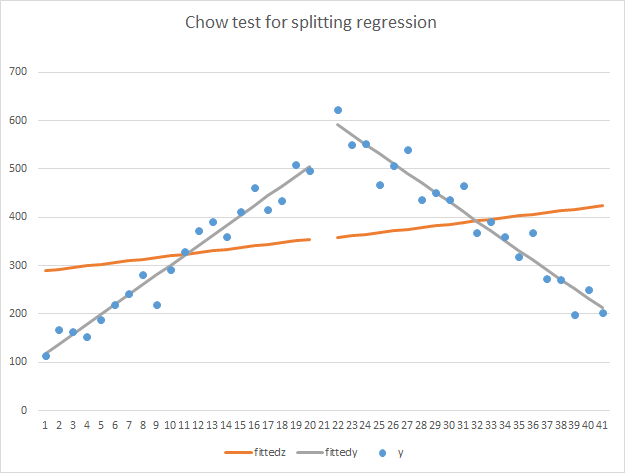17
Dec 16

## Testing for structural changes: a topic suitable for AP Stats

Testing for structural changes: a topic suitable for AP Stats

### Problem statement

Economic data are volatile but sometimes changes in them look more permanent than transitory.Figure 1. US GDP from agriculture. Source: http://www.tradingeconomics.com/united-states/gdp-from-agriculture

Figure 1 shows fluctuations of US GDP from agriculture. There have been ups and downs throughout the period of 2005-2016 but overall the trend has been up until 2013 and down since then. We want an objective, statistical confirmation of the fact that in 2013 the change was structural, substantial rather than a random fluctuation.

### Chow test steps

1. Divide the observed sample in two parts, A and B, at the point where you suspect the structural change (or break) has occurred. Run three regressions: one for A, another for B and the third one for the whole sample (pooled regression). Get residual sums of squares from each of them, denoted$RSS_A$$RSS_B$ and$RSS_p$, respectively.
2. Let$n_A$ and$n_B$ be the numbers of observations in the two subsamples and suppose there are$k$ coefficients in your regression (for Figure 1, we would regress GDP on a time variable, so the number of coefficients would be 2, including the intercept). The Chow test statistic is defined by$F_{k,n_A+n_B-2k}=\frac{(RSS_p-RSS_A-RSS_B)/k}{(RSS_A+RSS_B)/(n_A+n_B-2k)}.$

This statistic is distributed as$F$ with$k,n_A+n_B-2k$ degrees of freedom. The null hypothesis is that the coefficients are the same for the two subsamples and the alternative is that they are not. If the statistic is larger than the critical value at your chosen level of significance, splitting the sample in two is beneficial (better describes the data). If the statistic is not larger than the critical value, the pooled regression better describes the data.Figure 2. Splitting is better (there is a structural change)

In Figure 2, the gray lines are the fitted lines for the two subsamples. They fit the data much better than the orange line (the fitted line for the whole sample).Figure 3. Pooling is better

In Figure 3, pooling is better because the intercept and slope are about the same and pooling amounts to increasing the sample size.

Download the Excel file used for simulation. See the video.